### NCERT Solutions For Class 9 Math Chapter – 6 EXERCISE – 6.3

NCERT Solutions For Class 9 Math Chapter- 6 Exercise – 6.3

1. In Fig. 6.39, sides QP and RQ of ΔPQR are produced to points S and T respectively. If SPR = 135° and PQT = 110°, find PRQ.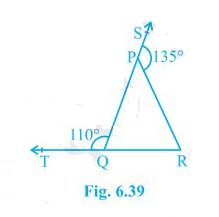Solution:

It is given the TQR is a straight line and so, the linear pairs (i.e. TQP and PQR) will add up to 180°

So, TQP +PQR = 180°

Now, putting the value of TQP = 110° we get,

PQR = 70°

Consider the ΔPQR,

Here, the side QP is extended to S and so, SPR forms the exterior angle.

Thus, SPR (SPR = 135°) is equal to the sum of interior opposite angles. (Triangle property)

Or,

PQR +PRQ = 135°

Now, putting the value of PQR = 70° we get,

PRQ = 135°-70°

Hence, PRQ = 65°

1. In Fig. 6.40, X = 62°, XYZ = 54°. If YO and ZO are the bisectors of XYZ and XZY respectively of Δ XYZ, find OZY and YOZ.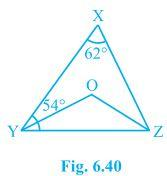Solution:

We know that the sum of the interior angles of the triangle.

So, X +XYZ +XZY = 180°

Putting the values as given in the question we get,

62°+54° +XZY = 180°

Or, XZY = 64°

Now, we know that ZO is the bisector so,

OZY = ½ XZY

∴ OZY = 32°

Similarly, YO is a bisector and so,

OYZ = ½ XYZ

Or, OYZ = 27° (As XYZ = 54°)

Now, as the sum of the interior angles of the triangle,

OZY +OYZ +O = 180°

Putting their respective values, we get,

O = 180°-32°-27°

Hence, O = 121°

1. In Fig. 6.41, if AB DE, BAC = 35° and CDE = 53°, find DCE.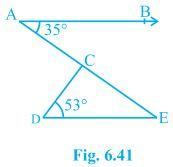Solution:

We know that AE is a transversal since AB DE

Here BAC and AED are alternate interior angles.

Hence, BAC = AED

It is given that BAC = 35°

AED = 35°

Now consider the triangle CDE. We know that the sum of the interior angles of a triangle is 180°.

∴ DCE+CED+CDE = 180°

Putting the values, we get

DCE+35°+53° = 180°

Hence, DCE = 92°

1. In Fig. 6.42, if lines PQ and RS intersect at point T, such that PRT = 40°, RPT = 95° and TSQ = 75°, find SQT.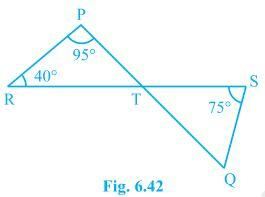Solution:

Consider triangle PRT.

PRT +RPT + PTR = 180°

So, PTR = 45°

Now PTR will be equal to STQ as they are vertically opposite angles.

So, PTR = STQ = 45°

Again, in triangle STQ,

TSQ +PTR + SQT = 180°

Solving this we get,

SQT = 60°

1. In Fig. 6.43, if PQ ⊥ PS, PQ SR, SQR = 28° and QRT = 65°, then find the values of x and y.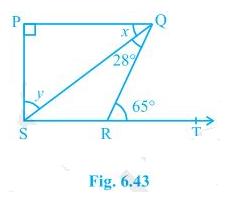Solution:

x +SQR = QRT (As they are alternate angles since QR is transversal)

So, x+28° = 65°

∴ x = 37°

It is also known that alternate interior angles are same and so,

QSR = x = 37°

Also, Now,

QRS +QRT = 180° (As they are a Linear pair)

Or, QRS+65° = 180°

So, QRS = 115°

Now, we know that the sum of the angles in a quadrilateral is 360°. So,

P +Q+R+S = 360°

Putting their respective values, we get,

S = 360°-90°-65°-115°

In Δ SPQ

∠SPQ + x + y = 1800

900 + 370 + y = 1800

y = 1800 – 1270 = 530

Hence, y = 53°

1. In Fig. 6.44, the side QR of ΔPQR is produced to a point S. If the bisectors of PQR and PRS meet at point T, then prove that QTR = ½ QPR.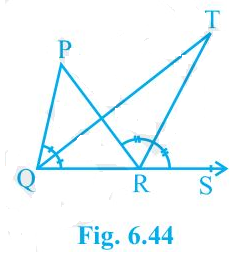Solution:

Consider the ΔPQR. PRS is the exterior angle and QPR and PQR are interior angles.

So, PRS = QPR+PQR (According to triangle property)

Or, PRS -PQR = QPR ———–(i)

Now, consider the ΔQRT,

TRS = TQR+QTR

Or, QTR = TRS-TQR

We know that QT and RT bisect PQR and PRS respectively.

So, PRS = 2 TRS and PQR = 2TQR

Now, QTR = ½ PRS – ½PQR

Or, QTR = ½ (PRS -PQR)

From (i) we know that PRS -PQR = QPR

So, QTR = ½ QPR (hence proved).